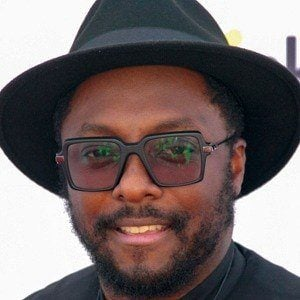# will.i.am Pop Singer

#1268
Most Popular
15, 1975
###### Birthplace
Los Angeles , California
43 years old
Pisces
1, 2003 (age 14)
###### Birthplace
Beverly Hills, CA

### Popularity

B o r n W i l l i a m J a m e s A d a m s , h e b e c a m e k n o w n f o r h i s w o r k w i t h t h e < a h r e f = ' / b a n d s / b l a c k - e y e d - p e a s ' t i t l e = ' B l a c k E y e d P e a s ' > B l a c k E y e d P e a s < / a > a l o n g s i d e < a h r e f = ' / s t a c y - f e r g u s o n ' t i t l e = ' F e r g i e ' > F e r g i e < / a > , < a h r e f = ' / j a i m e - g o m e z ' t i t l e = ' J a i m e G o m e z ' > J a i m e G o m e z < / a > a n d A l l a n P i n e d a . W i t h t h e < a h r e f = ' / b a n d s / b l a c k - e y e d - p e a s ' t i t l e = ' B l a c k E y e d P e a s ' > B l a c k E y e d P e a s < / a > , h e w o n s e v e n G r a m m y A w a r d s a n d t h r e e W o r l d M u s i c A w a r d s .

## will.i.am Before Fame

He was raised in the Boyle Heights housing project in East Los Angeles and attended Palisades Charter High School, where he befriended Allan Pineda , another future member of the Black Eyed Peas.

## will.i.am Trivial Things

He appeared in The Urbz: Sims In The City, which used The Black Eyed Peas' songs for its soundtrack. He won a Grammy Award for Best Rap Performance for "Let's Get It Started" in 2005.

## will.i.am Family Life

He was raised in Los Angeles, California by his mother Debra Cain.

## will.i.am Associated With

He wrote the song titled "Yes We Can," which supported the 2008 Barack Obama presidential campaign.